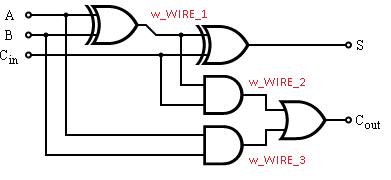Full Adder Module in VHDL and Verilog

Full adders are a basic building block for new digital designers. Lots of introductory courses in digital design present full adders to beginners. Once you understand how a full adder works, you can see how more complicated circuits can be built using only simple gates. I just want to make it clear to someone new that in reality, FPGA designers are not coding full adders by hand. The tools are advanced enough to know how to add two numbers together. It's still a good exercise, which is why it is presented here.

A single full-adder has two one-bit inputs, a carry-in input, a sum output, and a carry-out output. Many of them can be used together to create a ripple carry adder which can be used to add large numbers together. A single full-adder is shown in the picture below.1-bit Full-Adder Block - From Wikipedia

The next picture shows the entire schematic of the full adder and its corresponding truth table. The red text ties into the code below. w_WIRE_1, w_WIRE_2, w_WIRE_3 are the intermediate signals shown in the red text on the schematic.

Full Adder Truth Table
A B Cin Cout Sum
0 0 0 0 0
1 0 0 0 1
0 1 0 0 1
1 1 0 1 0
0 0 1 0 1
1 0 1 1 0
0 1 1 1 0
1 1 1 1 11-bit Full-Adder Detailed Schematic - Modified From Wikipedia

VHDL Implementation:

-------------------------------------------------------------------------------
-------------------------------------------------------------------------------
library ieee;
use ieee.std_logic_1164.all;
use ieee.numeric_std.all;

port (
i_bit1  : in std_logic;
i_bit2  : in std_logic;
i_carry : in std_logic;
--
o_sum   : out std_logic;
o_carry : out std_logic
);

architecture rtl of full_adder is

signal w_WIRE_1 : std_logic;
signal w_WIRE_2 : std_logic;
signal w_WIRE_3 : std_logic;

begin

w_WIRE_1 <= i_bit1 xor i_bit2;
w_WIRE_2 <= w_WIRE_1 and i_carry;
w_WIRE_3 <= i_bit1 and i_bit2;

o_sum   <= w_WIRE_1 xor i_carry;
o_carry <= w_WIRE_2 or w_WIRE_3;

-- FYI: Code above using wires will produce the same results as:
-- o_sum   <= i_bit1 xor i_bit2 xor i_carry;
-- o_carry <= (i_bit1 xor i_bit2) and i_carry) or (i_bit1 and i_bit2);

-- Wires are just used to be explicit.

end rtl;

Verilog Implementation:

///////////////////////////////////////////////////////////////////////////////
///////////////////////////////////////////////////////////////////////////////
(
i_bit1,
i_bit2,
i_carry,
o_sum,
o_carry
);

input  i_bit1;
input  i_bit2;
input  i_carry;
output o_sum;
output o_carry;

wire   w_WIRE_1;
wire   w_WIRE_2;
wire   w_WIRE_3;

assign w_WIRE_1 = i_bit1 ^ i_bit2;
assign w_WIRE_2 = w_WIRE_1 & i_carry;
assign w_WIRE_3 = i_bit1 & i_bit2;

assign o_sum   = w_WIRE_1 ^ i_carry;
assign o_carry = w_WIRE_2 | w_WIRE_3;

// FYI: Code above using wires will produce the same results as:
// assign o_sum   = i_bit1 ^ i_bit2 ^ i_carry;
// assign o_carry = (i_bit1 ^ i_bit2) & i_carry) | (i_bit1 & i_bit2);

// Wires are just used to be explicit.